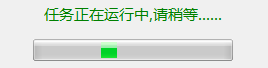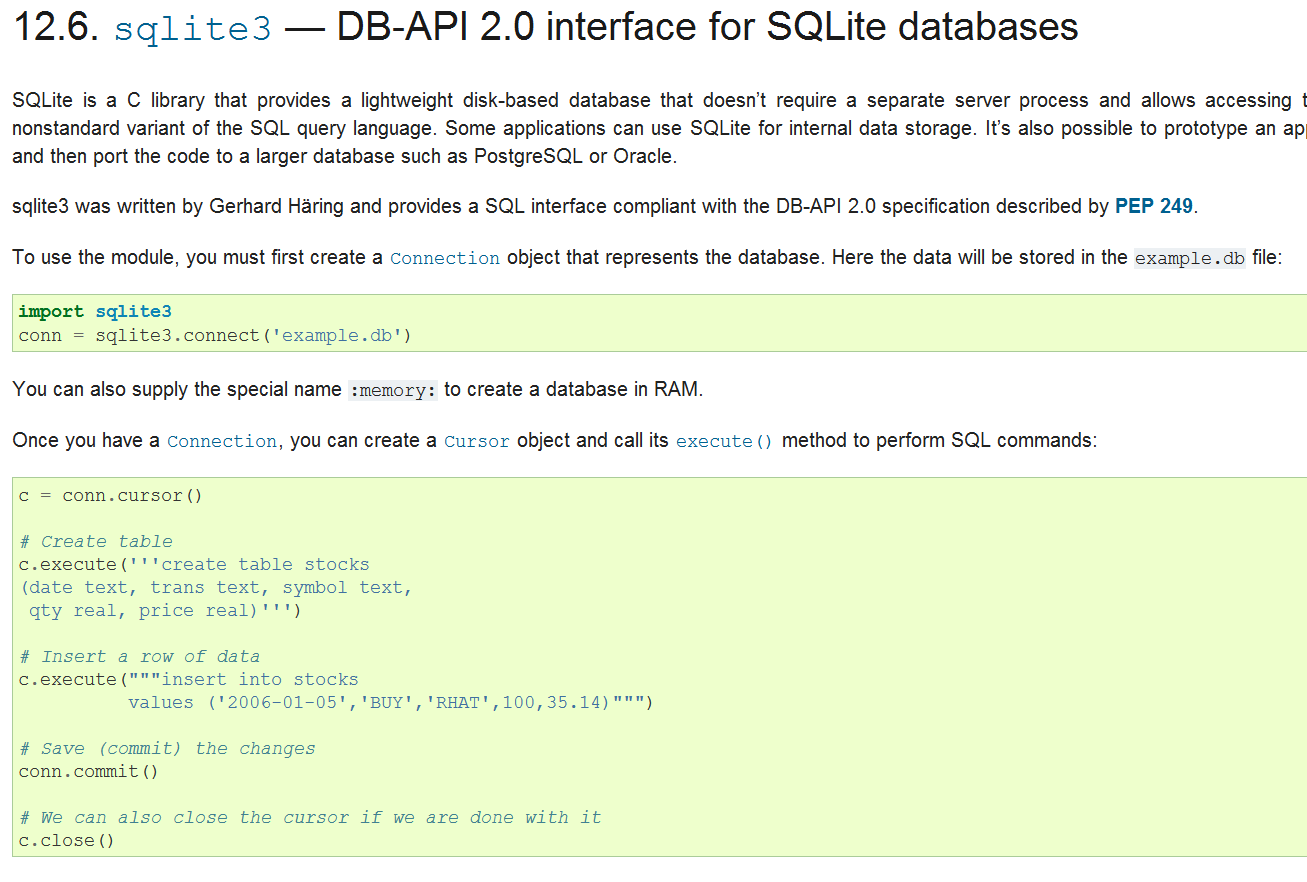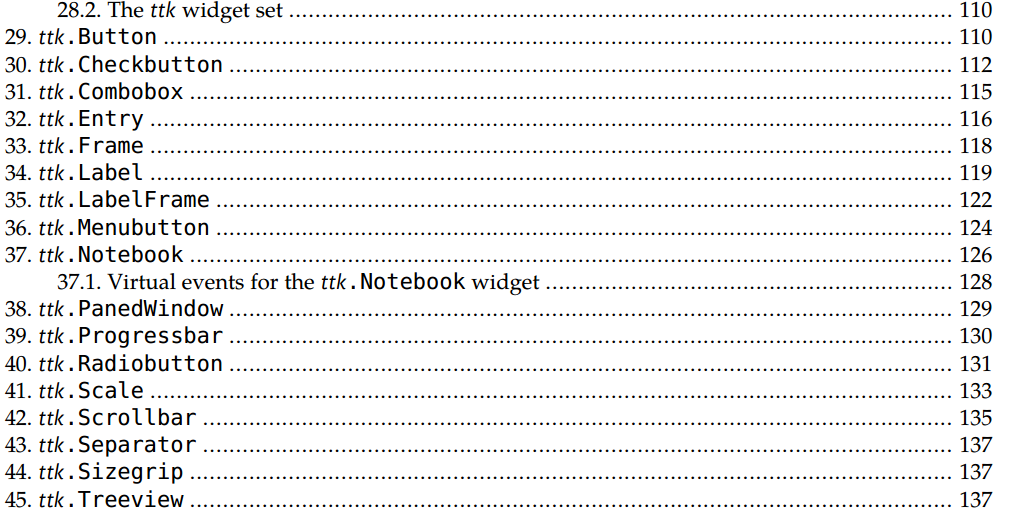# Python GUI之tkinter 实战（二）tkinter+多线程

## Python3 tkinter系列

### 自定义对话框

 Toplevel 顶层 类似框架,为其他的控件提供单独的容器

from tkinter import *
from tkinter import ttk

class GressBar():

def start(self):
top = Toplevel()
self.master = top
top.overrideredirect(True)
top.title("进度条")
prog = ttk.Progressbar(top, mode='indeterminate', length=200)
prog.start()

top.resizable(False, False)
top.update()
curWidth = top.winfo_width()
curHeight = top.winfo_height()
scnWidth, scnHeight = top.maxsize()
tmpcnf = '+%d+%d' % ((scnWidth - curWidth) / 2, (scnHeight - curHeight) / 2)
top.geometry(tmpcnf)
top.mainloop()

def quit(self):
if self.master:
self.master.destroy()### tkinter的UI线程与异步线程

a = input('请输入：')
print(a)


while 1:
a = input('请输入：')
print(a)


Ok，让我们再次运行上述代码，终于可以实现随时待命的程序了！但是有一点不爽，那就是当我们不想玩了的时候，我们似乎没法退出，当然我指的可不是关电脑！这就好像一个界面程序，他的边框上没有那个小×按钮，这真是让人郁闷难受，我们必须要加上这个×按钮，当然，命令行是没有按钮的，命令行通常用q命令或者exit退出，我们权且把q命令当做×按钮吧

while 1:
a = input('请输入：')
if a == 'q':
break
print(a)


from tkinter import *
from tkinter import ttk
import tkinter.filedialog as dir
import progressbar
import queue

class AppUI():

def __init__(self):
#创建一个消息队列
self.notify_queue = queue.Queue()
root = Tk()
self.master = root
self.create_content(root)
self.path = 'C:'
root.title("磁盘文件搜索工具")
………省略………
#创建一个进度条对话框实例
self.gress_bar = progressbar.GressBar()
#在UI线程启动消息队列循环
self.process_msg()
root.mainloop()

def process_msg(self):
self.top.after(400,self.process_msg)
while not self.notify_queue.empty():
try:
msg = self.notify_queue.get()
if msg == 1:
self.gress_bar.quit()

except queue.Empty:
pass


process_msg方法的最后，也就是对队列的操作了，其实Queue的方法也都很好理解，通过方法名我们大致都知道是什么意思了。这里首先通过empty方法判断一下队列是否为空，如果不为空就进入while循环，然后就从队列中取出第一条消息msg，此处我们定义往消息队列中存入元组，元组第一个元素用来表示消息类型，这里判断，如果消息类型为1，就关闭进度条对话框，执行完了这一条消息，就继续判断队列是否仍有消息，如果有就继续从消息队列里面取出第二条消息执行，若无消息则退出while循环。这样我们就在主线程建立了一个消息队列，每隔一段时间去消息队列里看看，有没有什么消息是需要主线程去做的，有一点需要特别注意，主线程消息队列里也不要干耗时操作，该队列仅仅用来更新UI。

    def execute_asyn(self):
#定义一个scan函数，放入线程中去执行耗时扫描
def scan(_queue):
pass
_queue.put((1,))

th.setDaemon(True)
th.start()
#启动进度条
self.gress_bar.start()


 file_menu.add_command(label="扫描",command=self.execute_asyn)


	#定义一个scan函数，放入线程中去执行耗时扫描
def scan(_queue):
time.sleep(2) #让线程睡眠2秒
_queue.put((1,))


### 实现磁盘文件快速搜索

import os
import os.path as pt

def scan_file(path):
result = []
for root,dirs,files in os.walk(path):
for f in files:
file_path = pt.abspath(pt.join(root,f))

result.append((file_path,file_path)) #保存路径与盘符

return result


os.walk函数用来遍历一个给定路径下的所有文件，通常这种磁盘文件遍历，都需要写递归实现，但是Python就是这么的强大，直接提供了这样一个生成器函数，从这一个个小点就可以看出，写Python代码能省多少事，多少心！

Python中还有很多类似的用一句话表达一些逻辑的语法糖，可自行体会

def scan_file(path):
return [(pt.abspath(pt.join(root,f)),pt.abspath(pt.join(root,f))) for root,dirs,files in os.walk(path) for f in files]



import sqlite3

class DataMgr():

def __init__(self):
#创建或打开一个数据库
#创建表
self.conn.execute('create table if not exists disk_table('\
'id integer primary key autoincrement,'\
'file_path text,'\
'drive_letter text)')
#创建索引，主要用来提高搜索速度
self.conn.execute('create index if not exists index_path on disk_table (file_path)')

#批量插入数据
def batch_insert(self,data):
for line in data:
self.conn.execute("insert into disk_table values (null,?,?)",line)

self.conn.commit()

#模糊搜索
def query(self,key):
cursor = self.conn.cursor()

cursor.execute("select file_path from disk_table where file_path like ?",('%{0}%'.format(key),))
r = [row for row in cursor]
cursor.close()
return r

def close(self):
self.conn.close()def execute_asyn(self):
#定义一个scan函数，放入线程中去执行耗时扫描
def scan(_queue):
if self.path:
paths = disk.scan_file(self.path)
self.data_mgr.batch_insert(paths)

_queue.put((1,))

th.setDaemon(True)
th.start()
#启动进度条
self.gress_bar.start()

def search_file(self):
if self.search_key.get():
result_data = self.data_mgr.query(self.search_key.get())
if result_data:
self.list_val.set(tuple(result_data))from tkinter import *
from tkinter import ttk
import tkinter.filedialog as dir
from database import DataMgr

class AppUI():

def __init__(self):
self.notify_queue = queue.Queue()
root = Tk()
self.master = root
self.create_content(root)
self.path = 'C:'
root.title("磁盘文件搜索工具")
root.update()
# root.resizable(False, False) 调用方法会禁止根窗体改变大小
#以下方法用来计算并设置窗体显示时，在屏幕中心居中
curWidth = root.winfo_width()
curHeight = root.winfo_height()
scnWidth, scnHeight = root.maxsize()
tmpcnf = '+%d+%d' % ((scnWidth - curWidth) / 2, (scnHeight - curHeight) / 2)
root.geometry(tmpcnf)

self.gress_bar = progressbar.GressBar()
self.data_mgr = DataMgr()
self.process_msg()
root.mainloop()

#创建菜单栏

# 创建二级菜单

#在菜单栏中添加菜单

def create_content(self, root):
lf = ttk.LabelFrame(root, text="文件搜索")

top_frame = Frame(lf)

self.search_key = StringVar()
ttk.Entry(top_frame, textvariable=self.search_key,width=50).pack(fill=X,expand=YES,side=LEFT)

bottom_frame = Frame(lf)

band = Frame(bottom_frame)
band.pack(fill=BOTH,expand=YES,side=TOP)

self.list_val = StringVar()
listbox = Listbox(band,listvariable=self.list_val,height=18)
listbox.pack(side=LEFT,fill=X,expand=YES)

vertical_bar = ttk.Scrollbar(band,orient=VERTICAL,command=listbox.yview)
vertical_bar.pack(side=RIGHT,fill=Y)
listbox['yscrollcommand'] = vertical_bar.set

horizontal_bar = ttk.Scrollbar(bottom_frame,orient=HORIZONTAL,command=listbox.xview)
horizontal_bar.pack(side=BOTTOM,fill=X)
listbox['xscrollcommand'] = horizontal_bar.set

#给list动态设置数据，set方法传入一个元组，注意此处是设置，不是插入数据，此方法调用后，list之前的数据会被清除
self.list_val.set(('jioj',124,"fjoweghpw",1,2,3,4,5,6))

def process_msg(self):
self.master.after(400,self.process_msg)
while not self.notify_queue.empty():
try:
msg = self.notify_queue.get()
if msg == 1:
self.gress_bar.quit()

except queue.Empty:
pass

def execute_asyn(self):
#定义一个scan函数，放入线程中去执行耗时扫描
def scan(_queue):
if self.path:
paths = disk.scan_file(self.path)
self.data_mgr.batch_insert(paths)

_queue.put((1,))

th.setDaemon(True)
th.start()
#启动进度条
self.gress_bar.start()

def search_file(self):
if self.search_key.get():
result_data = self.data_mgr.query(self.search_key.get())
if result_data:
self.list_val.set(tuple(result_data))

def open_dir(self):
d = dir.Directory()
self.path = d.show(initialdir=self.path)

if __name__ == "__main__":
AppUI()


12-193万+